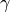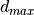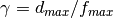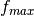Minimize Energy : A Component of the Hierarchical Equilibration Strategy for Polymer Melts¶

The module is an implementation of a part of a hierarchical strategy  for the equilibration of simple one-component polymer melts in ESPResSO++.

Purpose of Module¶

To study the properties of polymer melts by numerical simulations, equilibrated configurations must be prepared. However, the relaxation time for high molecular weight polymer melts is huge and increases, according to reptation theory, with the third power of the molecular weight. Hence, an effective method for decreasing the equilibration time is required. The hierarchical strategy pioneered in Ref.  is a particularly suitable way to do this. The present module provides a part of that method.

When the microscopic monomers are re-inserted into the soft blobs, the polymer configurations should satisfy a local energy minimum to avoid overlap between particles. This module provides a steepest-descent method <https://en.wikipedia.org/wiki/Gradient_descent>`_ which is a typical energy minimization method.

This module is a modification of the already existing class espressopp.integrator.MinimizeEnergy. The modifications are as follows:

1. Corrected the procedure of particle redistribution to cells.
2. A variable relaxation of the energy per stepis implemented. In this case, the position of particles is updated following the equation: .. math:: p_{i+1} = p_i + (d_{max}/f_{max}) F_i whereis the maximum update of particle coordinates in a single steepest-descent step andis adjusted viawhereis the maximum force in a single step.

These modifications significantly stabilize the procedure of redistributing particles to cells, and any valueless than half the skin parameter of the Verlet list can be used.

Background Information¶

The implementation of this module is based on ESPResSO++. You can learn about ESPResSO++ from the following links:

Testing¶

Explanation of installation:

After installing this module, it can be tested by a Python script found under the following link:

Source Code¶

This module has been merged into ESPResSo++: